Next: 13.2.2 Pixel Scale and Up: 13.2 Colors and Color Previous: 13.2 Colors and Color   Contents

### 13.2.1 The Accumulation Buffer: Interpolation and Extrapolation

Haeberli and Voorhies  have suggested several interesting image processing techniques using linear interpolation and extrapolation. Each technique is stated in terms of the formula: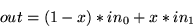(12)

This equation is evaluated on a per-pixel basis.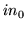and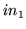are the input images,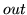is the output image, and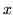is the blending factor. Ifis between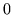and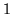, the equations describe a linear interpolation. Ifis allowed to range outside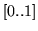, the result is extrapolation .

In the limited case where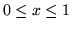, these equations may be implemented using the accumulation buffer via the following steps:

38.
Drawinto the color buffer.
39.
Load, scaling by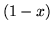(glAccumGL_ LOAD, (1-x)(GL_ LOAD, (1-x))).
40.
Drawinto the color buffer.
41.
Accumulate, scaling by(glAccumGL_ ACCUM,x(GL_ ACCUM,x)).
42.
Return the results (glAccumGL_ RETURN, 1(GL_ RETURN, 1)).
It is assumed thatandare betweenand. Since the accumulation buffer can only store values in the range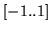, for the case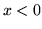or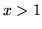, the equation must be implemented in a different way. Given the value, you can modify equation 12 and derive a list of accumulation buffer operations to perform the operation. Define a scale factor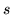such that: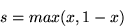Equation 12 becomes: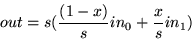and the list of steps becomes:
43.
Compute.
44.
Drawinto the color buffer.
45.
Load, scaling by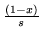(glAccumGL_ LOAD, (1-x)/s(GL_ LOAD, (1-x)/s)).
46.
Drawinto the color buffer.
47.
Accumulate, scaling by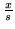(glAccumGL_ ACCUM, x/s(GL_ ACCUM, x/s)).
48.
Return the results, scaling by(glAccumGL_ RETURN, s(GL_ RETURN, s)).

The techniques suggested by Haeberli and Voorhies use a degenerate image asand an appropriate value ofto move toward or away from that image. To increase brightness,is set to a black image and. To change contrast,is set to a gray image of the average luminance value of. Decreasing(toward the gray image) decreases contrast; increasingincreases contrast. Saturation may be varied using a luminance version ofas. (For information on converting RGB images to luminance, see Section 13.2.4.) Sharpening may be accomplished by settingto a blurred version of.Next: 13.2.2 Pixel Scale and Up: 13.2 Colors and Color Previous: 13.2 Colors and Color   Contents
2001-01-10